Relationships among Estimation Criteria

There is always some arbitrariness to classify the estimation methods according to certain mathematical or numerical properties. The discussion in this section is not meant to be a thorough classification of the estimation methods available in PROC CALIS. Rather, classification is done here with the purpose of clarifying the uses of different estimation methods and the theoretical relationships of estimation criteria.

### Assumption of Multivariate Normality

GLS, ML, and FIML assume multivariate normality of the data, while ULS, WLS, and DWLS do not. Although the ML method with covariance structure analysis alone can also be based on the Wishart distribution of the sample covariance matrix, for convenience GLS, ML, and FIML are usually classified as normal-theory based methods, while ULS, WLS, and DWLS are usually classified as distribution-free methods.

An intuitive or even naive notion is usually that methods without distributional assumptions such as WLS and DWLS are preferred to normal theory methods such as ML and GLS in practical situations where multivariate normality is doubt. This notion might need some qualifications because there are simply more factors to consider in judging the quality of estimation methods in practice. For example, the WLS method might need a very large sample size to enjoy its purported asymptotic properties, while the ML might be robust against the violation of multi-normality assumption under certain circumstances. No recommendations regarding which estimation criterion should be used are attempted here, but you should make your choice based more than the assumption of multivariate normality.

### Contribution of the Off-Diagonal Elements to the Estimation of Covariance or Correlation Structures

If only the covariance or correlation structures are considered, the six estimation functions,
,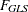,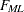,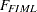,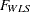, and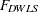, belong to the following two groups:

• The functions,,, andtake into account all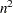elements of the symmetric residual matrix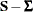. This means that the off-diagonal residuals contribute twice to the discrepancy function, as lower and as upper triangle elements.

• The functionsandtake into account only the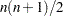lower triangular elements of the symmetric residual matrix. This means that the off-diagonal residuals contribute to the discrepancy functiononly once.

Thefunction used in PROC CALIS differs from that used by the LISREL 7 program. Formula (1.25) of the LISREL 7 manual (Jöreskog and Sörbom; 1985, p. 23) shows that LISREL groups thefunction in the first group by taking into account allelements of the symmetric residual matrix.

• Relationship between DWLS and WLS:
PROC CALIS: Theanddiscrepancy functions deliver the same results for the special case that the weight matrix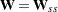used by WLS estimation is a diagonal matrix.
LISREL 7: This is not the case.

• Relationship between DWLS and ULS:
LISREL 7: Theandestimation functions deliver the same results for the special case that the diagonal weight matrixused by DWLS estimation is an identity matrix.
PROC CALIS: To obtain the same results withandestimation, set the diagonal weight matrixused in DWLS estimation to: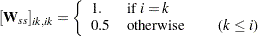Because the reciprocal elements of the weight matrix are used in the discrepancy function, the off-diagonal residuals are weighted by a factor of 2.

### ML and FIML Methods

Both the ML and FIML methods can be derived from the log-likelihood function for multivariate normal data. The preceding section Estimation Criteria mentions thatis essentially the same as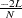, where
is the log-likelihood function for multivariate normal data. For the ML estimation, you can also consideras a part of thediscrepancy function that contains the information regarding the model parameters (while the rest thefunction contains some constant terms given the data). That is, with some algebraic manipulations and assuming that there is no missing value in the analysis (so that all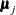and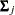are the same as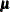and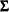, respectively), it can shown that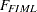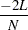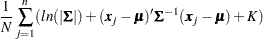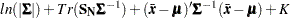where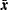is the sample mean and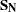is the biased sample covariance matrix. Compare this FIML function with the ML function shown in the following expression, which shows that both functions are very similar: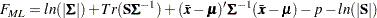The two expressions differ only in the constant terms, which are independent of the model parameters, and in the formulas for computing the sample covariance matrix. While the FIML method assumes the biased formula (with
as the divisor, by default) for the sample covariance matrix, the ML method (as implemented in PROC CALIS) uses the unbiased formula (with
as the divisor, by default).

The similarity (or dissimilarity) of the ML and FIML discrepancy functions leads to some useful conclusions here:

• Because the constant terms in the discrepancy functions play no part in parameter estimation (except for shifting the function values), overriding the default ML method with VARDEF=N (that is, usingas the divisor in the covariance matrix formula) leads to the same estimation results as that of the FIML method, given that there are no missing values in the analysis.

• Because the FIML function is evaluated at the level of individual observations, it is much more expensive to compute than the ML function. As compared with ML estimation, FIML estimation takes longer and uses more computing resources. Hence, for data without missing values, the ML method should always be chosen over the FIML method.

• The advantage of the FIML method lies solely in its ability to handle data with random missing values. While the FIML method uses the information maximally from each observation, the ML method (as implemented in PROC CALIS) simply throws away any observations with at least one missing value. If it is important to use the information from observations with random missing values, the FIML method should be given consideration over the ML method.

See Example 26.14 for an application of the FIML method and Example 26.15 for an empirical comparison of the ML and FIML methods.